# Test: Class 12 Mathematics: CBSE Sample Question Paper- Term I (2021-22)

## 50 Questions MCQ Test Sample Papers for Class 12 Medical and Non-Medical | Test: Class 12 Mathematics: CBSE Sample Question Paper- Term I (2021-22)

Description
Attempt Test: Class 12 Mathematics: CBSE Sample Question Paper- Term I (2021-22) | 50 questions in 40 minutes | Mock test for JEE preparation | Free important questions MCQ to study Sample Papers for Class 12 Medical and Non-Medical for JEE Exam | Download free PDF with solutions
QUESTION: 1

### What is the principal value branch of cot–1x ?

Solution:

The cotangent function is periodic so to calculate its inverse function we need to make the function bijective. For that we have to consider an interval in which all values of the function exist and do not repeat. For cotangent function this interval is considered as (0, π).

Thus when we take the inverse of the function the domain becomes range and the range becomes domain. Hence the principal value branch is the range of cot–1 x that is (0, π).

QUESTION: 2

### What is the continuity of [x] at x = 3.5 ?

Solution:

L.H. L=R.H. L = f(x)

QUESTION: 3

### Choose the correct option for the given matrix.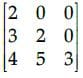Solution:

A square matrix is called lower triangular if all the entries above the main diagonal are zero. Similarly, a square matrix is called upper triangular if all the entries below the main diagonal is zero.
Hence, given matrix is lower triangular matrix.

QUESTION: 4

If a matrix has 4 rows and 3 columns then how many elements will be there in this matrix?

Solution:

Matrix is represented by m × n
Where m = no. of rows & n = no. of column
And number of total elements = mn
If a matrix has 4 rows and 3 columns hen the number of elements in the matrix is 12.

QUESTION: 5

What is the equation of the normal to the curve y = 3x2 – 7x + 5 at (0, 5)?

Solution:

Tangent and normal are perpendicular to each other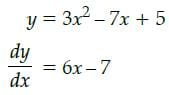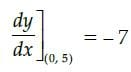∴ Slope of normal = 1/7
Equation of normal is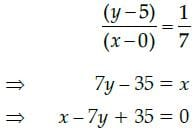QUESTION: 6

If A is a square matrix of order 3, such that A(adj A) = 10I, then |adj A| is equal to

Solution:

Consider the equation
Here, A(adj A) = 10 I
Then, |A| = 10
Where n is order of matrix
Here, = |A|3–1 = 102 = 100

QUESTION: 7

R be the relation in the set N given by R = {(a, b) : a = b –2, b > 6}. Then, the correct option is:

Solution:

Take b =8, ⇒ a = 6.
Hence, (6, 8) ∈ R

QUESTION: 8

Evaluate the determinant of the matrix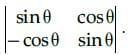Solution:

The determinant of a square matrix of order 2 is given by the difference of the product of diagonal elements and the product of the offdiagonal elements.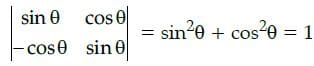QUESTION: 9

Which of the function is not differentiable everywhere in R?

Solution:

|x| is not differentiable at x=0

QUESTION: 10

All the trigonometric functions have inverse functions irrespective of the domain.

Solution:

For the inverse of a function to exist the function should be bijective which none of the trigonometric function is as they are periodic functions.

QUESTION: 11

Let R be the relation in the set {5, 6, 7, 8} given by R = {(5, 6), (6, 6), (5, 5), (8, 8), (5, 7), (7, 7), (7, 6)}.

Solution:

Let R be the relation in the set {1, 2,3, 4} is given by:
R = {(5,6), (6,6), (5,5), (8,8), (5,7), (7,7), (7,6)}
(a) (5,5), (6,6), (7,7), (8,8) ∈ R Therefore, R is reflexive.
(b) (5,6) ∈ R but (6,5) ∈ R. Therefore, R is not symmetric.
(c) If (5, 7) ∈ R and (7, 6) ∈ R then (5, 6) ∈ R. Therefore, R is transitive.

QUESTION: 12

What is the equation of the tangent to the curve y – 5x2 = 0 and parallel to line 5x + y + 1 = 0 ?

Solution:

Given equation of the curve is y – 5x2 = 0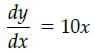Slope of the line 5x + y + 1 = 0 is – 5 slope of the tangent = slope of the parallel line
i.e., 10x = – 5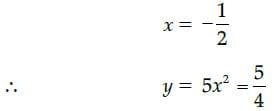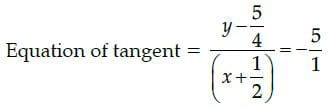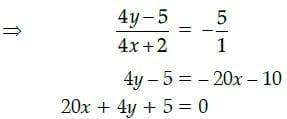QUESTION: 13

if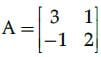then A2−5A + 7I2 is equal to :

Solution: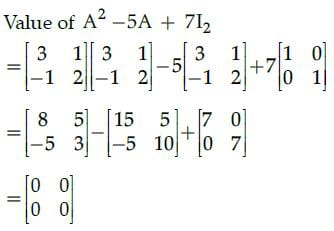Since, all the elements of matrix are zero. So, given matrix is null/zero matrix.

QUESTION: 14

If y = log(cosex) then dy/dx will be:

Solution: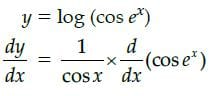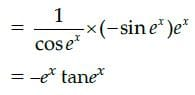QUESTION: 15

For a square matrix A = [aij] the quantity calculated for any element aij in A as the determinant of thesquare sub-matrix of order (n – 1) obtained by leaving the ith row and jth column of A is known as:

Solution:

The minor of an element aij in A is calculated as the determinant of the square sub-matrix of order (n-1) obtained by leaving the ith row and jth column of A.

QUESTION: 16

Which of the following is satisfied by the given function y = sin10x−cos10x ?

Solution: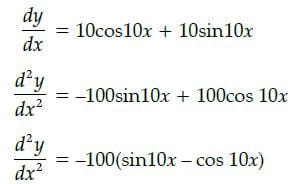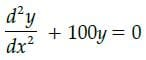QUESTION: 17

If matrix A = [2 3 5], then the value of A.A' is:

Solution:

A = [2 3 5]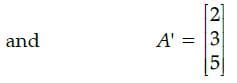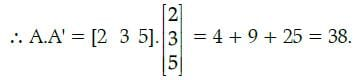QUESTION: 18

The function y = 5x2 – 32x has a local minimum in the interval (0,10).

Solution:

let f(x) = 5x2 – 32x
f '(x) = 10x – 32
10x – 32 = 0
f"(x) for x = 3.2 and f'(x)<0 for x >3.2 .

QUESTION: 19

Z = 30x1 + 30x2, subject to x1 ≥ 0, x2 ≥ 0, x1 + 3x2 ≤ 6, 4x1 + 8x2 ≥ 16, x1 + x2 ≤ 4. The minimum value of Z occurs at

Solution:

Corner Points are (0, 2), (2, 1), (4, 0) and (3, 1).
The objective function for (x, y) is 30x1 + 30x2.
After putting the corner points in the objective function, we get the minimum value of Z.

QUESTION: 20

A rectangle of maximum area is inscribed in a circle of radius R.Then which of the following is true?

Solution:

On applying Second Derivative test,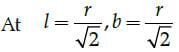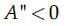QUESTION: 21

Let T be the set of all triangles in a plane with R is a relation in T given by R = {(T1, T2) : T1 is congruent to T2}. Then R is

Solution:

For reflexive, T1 is congruent to T1
⇒ (T1,T1) Î R For symmetric, (T1,T2) ∈ R ⇒ T1 is congruent to T2 ⇒ T2 is congruent to T1 ⇒ (T2,T1) ∈ R. Hence it is symmetric.
For transitive, (T1, T2) ∈ R ⇒ T1 is congruent to T2 and (T2,T3) ∈ R ⇒ T2 is congruent to T3 which implies T1 is congruent to T3 ⇒ (T1,T3) ∈ R. Hence, it is transitive.
Hence, R is an equivalence relation.

QUESTION: 22

Consider the function 3x4 +20x3 −36x2 +44 in the interval [−5,10] , function is maximum at

Solution:

Let f(x) = 3x4 + 20x3 – 36x2 + 44
f '(x) = 12x3 + 60x2 – 72x
f'(x) = 0
12x3 + 60x2 – 72x = 0
12x (x2 + 5x – 6) = 0
12x (x – 3) (x – 2) = 0
x = 0, 2, 3
f''(x) = 36x2 + 120x – 72
f''(0) = –72 < 0
f''(2) = 312 > 0
f''(3) = 612 > 0
∴ function has a maxima at x = 0

QUESTION: 23

The graph of x ≤ 2 and y ≥ 2 will be situated in quadrants.

Solution: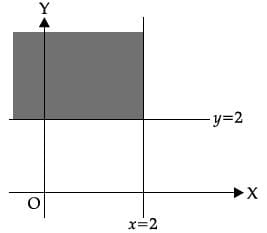QUESTION: 24

What is the value of l in the function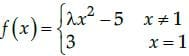provided function is continuous at x = 1?

Solution:

For a function to be continuous L.H. L=R.H. L= f (x)

QUESTION: 25

If A is a 3 × 3 matrix such that |A| = 8, then |3A| equals

Solution:

Here |A| = 8
Then |3A| = 33|A| = 27 × 8 = 216

QUESTION: 26

The function f(x) = tan x – x

Solution:

We have
f(x) = tan x – x
On differentiating with respect to x, we
get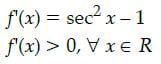So, f(x) always increases.

QUESTION: 27

What is the domain of the cot–1 x ?

Solution:

The cot function is periodic so to calculate its inverse function we need to make the function bijective. For that we have to consider an interval in which all values of the function exist and do not repeat. Now for the inverse of a function the domain becomes range and the range becomes domain. Thus the range of cot function, that is, (-∞, ∞) becomes the domain of inverse function.

QUESTION: 28

If A is skew symmetric matrix of order 3, then the value of |A| is

Solution:

Determinant value of skew symmetric matrix is always '0'.

QUESTION: 29

The function f(x) = 4sin3x – 6 sin2x + 12 sinx + 100 is strictly

Solution:

Given that, f(x) = 4sin3x – 6 sin2x + 12 sinx 100
On differentiating with respect to x, we get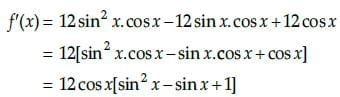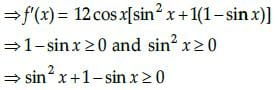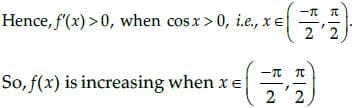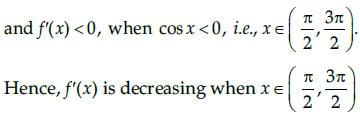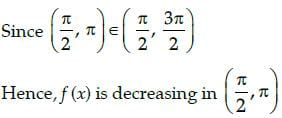QUESTION: 30

If A = {1, 2}, B = {3,4, 5} and f = {(1, 3), (2, 5)} is a function from A to B, then f(x) is

Solution:

Given, A = {1, 2}, B = {3,4, 5} and f : A → B is defined as f = {(1, 3), (2, 5)} i.e., f(1) = 3, f(2) = 5.
We can see that the images of distinct elements of A under f are distinct. So, f is one-one.

QUESTION: 31

The function f(x) = e|x| is

Solution:

Given that,
f (x) = e|x|

The functions ex and |x| are continuous functions for all real value of x. Since ex is differentiable everywhere but |x| is non-differentiable at x = 0.
Thus, the given functions f(x) = e|x| is continuous everywhere but not differentiable at x = 0.

QUESTION: 32

The matrix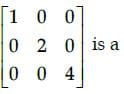Solution: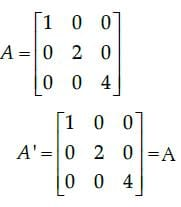So, the given matrix is a symmetric matrix. [Since, in a square matrix A, if A’ = A, then A is called symmetric matrix.]

QUESTION: 33

Z = 7x + y, subject to 5x + y £ 5, x + y ³ 3, x ³ 0, y ≥ 0. Maximum value of Z occurs at

Solution: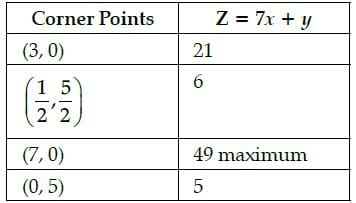QUESTION: 34

Which of the following functions is decreasing on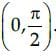Solution:

In the given interval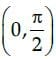f(x) = cos x
On differentiating with respect to x, we get f´(x) = – sin x
which gives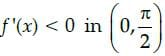Hence, f(x) = cosx is decreasing in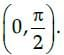QUESTION: 35

If A and B are symmetric matrices of same order, then AB – BA is a:

Solution:

A and B are symmetric matrices.
⇒ A = A’ and B = B’
Now, (AB – BA)’ = (AB)’ – (BA)’ ...(i)
⇒ (AB – BA)’=B’A’ – A’B’ [By reversal law]
⇒ (AB – BA)’ = BA – AB [From Eq. (i)]
⇒ (AB – BA)’ = –(AB – BA)
⇒ (AB – BA) is a skew-symmetric matrix.

QUESTION: 36

The principal value of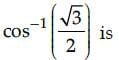Solution:

The principal value of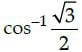means that we need to find an angle in the principal branch of the function where the cosine function is equal to √3/2 . Hence the required value
is π/6

QUESTION: 37

The domain of sin–1 x is :

Solution:

The domain of sin-1 x is [-1, 1].

QUESTION: 38

if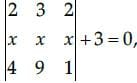then the value of x is

Solution: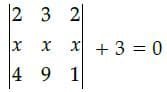On expanding along R1
2(x – 9x) – 3(x – 4x)+ 2(9x – 4x) + 3 = 0
2(–8x) – 3(–3x) + 2(5x) + 3 = 0
– 16x + 9x + 10x + 3 = 0
3x + 3 = 0
3x = –3
x = - 3/3
x = –1

QUESTION: 39

The contentment obtained after eating x units of a new dish at a trial function is given by the functionf(x) = x3 + 6x2 + 5x + 3. The marginal contentment when 3 units of dish are consumed is ____________.

Solution: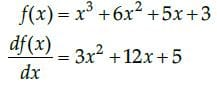At x = 3,
Marginal contentment
= 3x (3)2 + 12 x 3 + 5
= 27+ 36 +5
68 units.

QUESTION: 40

The matrix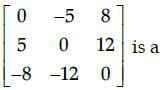Solution:

We know that, in a square matrix, if bij = 0 when i ≠ j then it is said to be a diagonal matrix. Here, b12, b13…. ≠ 0
so the given matrix is not a diagonal matrix.
Now,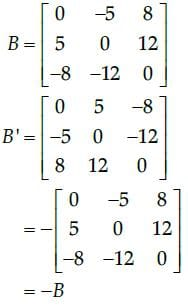So, the given matrix is a skew-symmetric matrix, since we know that in a square matrix B, if B’ = − B, then it is called skew-symmetric matrix.

QUESTION: 41

A dealer in rural area wishes to purchase a number of sewing machines. He has only ₹5,760 to invest and
has space for at most 20 items for storage. An electronic sewing machine cost him ₹360 and a manually
operated sewing machine ₹240. He can sell an electronic sewing machine at a profit of ₹22 and a manually
operated machine at a profit of ₹18. Assume that the electronic sewing machines he can sell is x and that
of manually operated machines is y.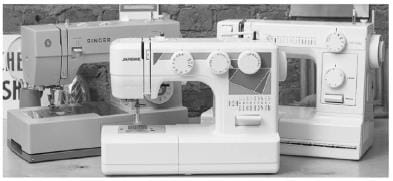The objective function is _______.

Solution:
QUESTION: 42

The maximum value of sin x. cos x is

Solution:

Let us assume that,
f(x) = sin x cos x
Now, we know that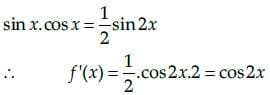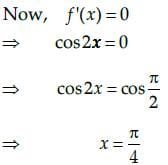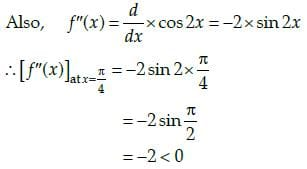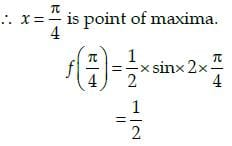QUESTION: 43

The maximum value of (1/x)is:

Solution: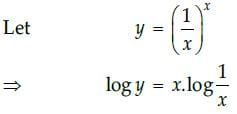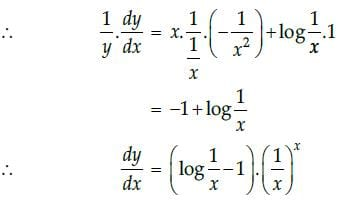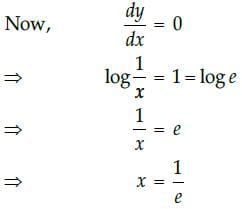Hence, the maximum value of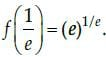QUESTION: 44

From the figure, which point is not belongs to feasible region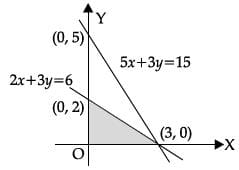Solution:
QUESTION: 45

Which of the given values of x and y make the following pair of matrices equal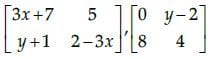Solution:

It is given that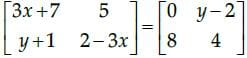Equating the corresponding elements, we get
3x + 7 = 0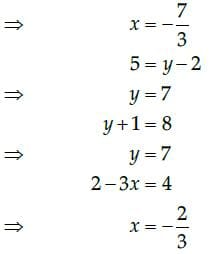We find that on comparing the corresponding elements of the two matrices, we get two different values of x, which is not possible.
Hence, it is not possible to find the values of x and y for which the given matrices are equal.

QUESTION: 46

Case - Study

The Relation between the height of the plant (y in cm) with respect to exposure to sunlight is governed
by the following equation y = 4x -1/2x2 . where x is the number of days exposed to sunlight.The rate of growth of the plant with respect to sunlight is ______ .

Solution: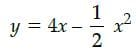∴ rate of growth of the plant with respect
to sunlightQUESTION: 47

Case - Study

The Relation between the height of the plant (y in cm) with respect to exposure to sunlight is governed
by the following equation y = 4x -1/2x2 . where x is the number of days exposed to sunlight.What is the number of days it will take for the plant to grow to the maximum height?

Solution: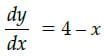The number of days it will take for the plant to grow to the maximum height,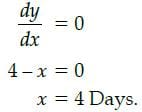QUESTION: 48

Case - Study

The Relation between the height of the plant (y in cm) with respect to exposure to sunlight is governed
by the following equation y = 4x -1/2x2 . where x is the number of days exposed to sunlight.What is the maximum height of the plant?

Solution:

We have, number of days for maximum height of plant = 4 Days
∴ Maximum height of plant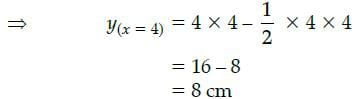QUESTION: 49

Case - Study

The Relation between the height of the plant (y in cm) with respect to exposure to sunlight is governed
by the following equation y = 4x -1/2x2 . where x is the number of days exposed to sunlight.What will be the height of the plant after 2 days?

Solution:

Height of plant after 2 days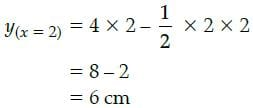QUESTION: 50

Case - Study

The Relation between the height of the plant (y in cm) with respect to exposure to sunlight is governed
by the following equation y = 4x -1/2x2 . where x is the number of days exposed to sunlight.If the height of the plant is 7/2 cm, the number of days it has been exposed to the sunlight is _____ .

Solution: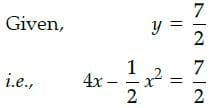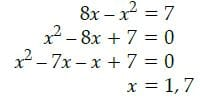We will take x = 1, because it will take 4 days for the plant to grow to the maximum height i.e. 8 cm and 7/2 cm is not maximum height so, it will take less than 4 days. i.e., 1 Day.Use Code STAYHOME200 and get INR 200 additional OFF Use Coupon Code